# RS Aggarwal Class 8 Solutions Chapter 10 Profit and Loss Ex 10B

## RS Aggarwal Class 8 Solutions Chapter 10 Profit and Loss Ex 10B

These Solutions are part of RS Aggarwal Solutions Class 8. Here we have given RS Aggarwal Solutions Class 8 Chapter 10 Profit and Loss Ex 10B.

Other Exercises

Question 1.
Solution:
Marked price of cooler = Rs 4650
Rate of discount = 18%
Selling price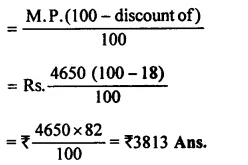Question 2.
Solution:
Marked price = Rs 960
Selling price = Rs 816
Total Discount = M.P. – S.P.
= Rs 960 – Rs 816
= Rs 144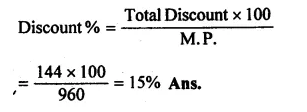Question 3.
Solution:
S.P. of shirt = Rs 1092
Discount = Rs 208
M.P. of shirt = S.P. + discountQuestion 4.
Solution:
S.P. of toy = Rs 216.20
Discount = 8%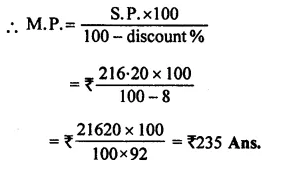Question 5.
Solution:
S.P. of tea set = Rs 528
Rate of discount = 12%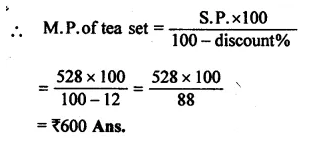Question 6.
Solution:
Let C.P. of goods = Rs 100
Marked price = Rs 100 + 35
= Rs 135
Rate of discount = 20%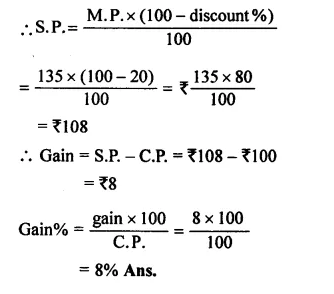Question 7.
Solution:
Let C.P. of phone = Rs 100
.’. Marked price = Rs 100 + 40
= Rs 140
Rate of discount = 30%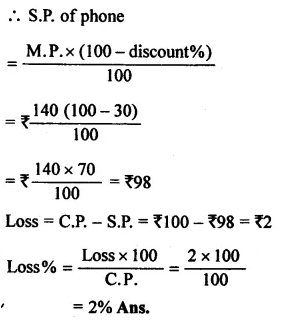Question 8.
Solution:
C.P. of fan = Rs. 1080
Gain = 25%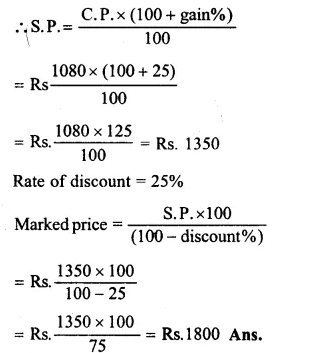Question 9.
Solution:
C.P of refrigerator = Rs. 11515
and gain % = 20%
S.P. of refrigerator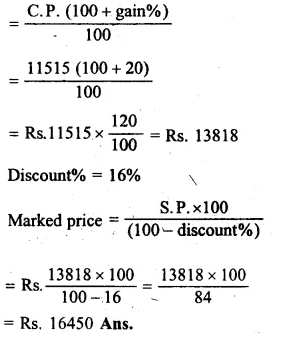Question 10.
Solution:
C.P. of ring = Rs. 1190
Gain = 20%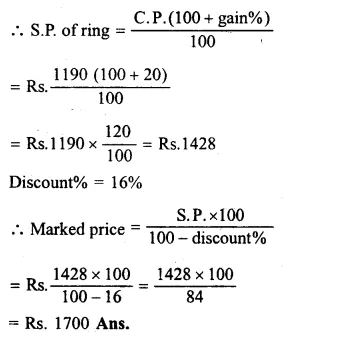Question 11.
Solution:
Let Marked price = Rs. 100
Discount = 10%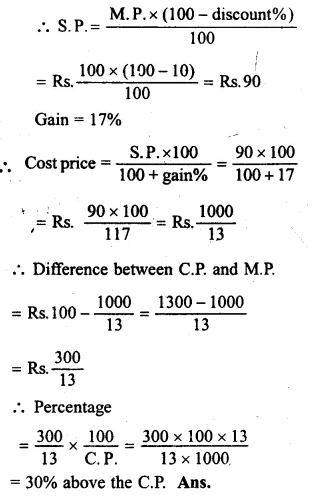Question 12.
Solution:
Let C.P. = Rs. 100
Gain = 8%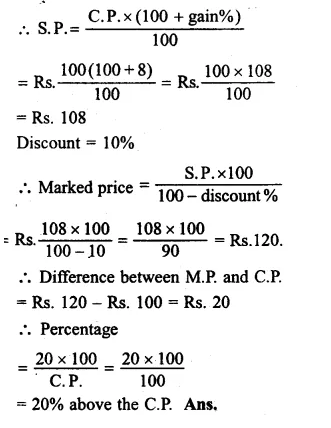Question 13.
Solution:
Marked price of TV = Rs. 18500
Series of two successive discounts = 20% and 5%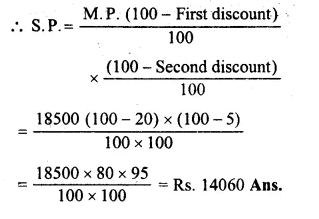Question 14.
Solution:
Let M.P. = Rs. 100
First discount = 20%
and second discount = 5%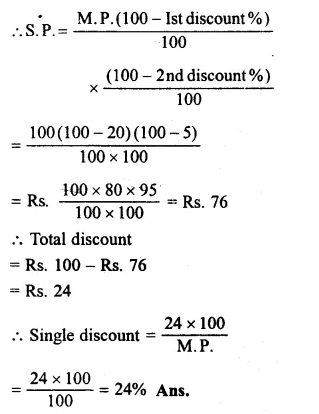Hope given RS Aggarwal Solutions Class 8 Chapter 10 Profit and Loss Ex 10B are helpful to complete your math homework.

If you have any doubts, please comment below. Learn Insta try to provide online math tutoring for you.